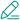#臭大佬

### PHP生成不重复随机数的方法汇总

本文汇总了5种生成不重复随机数的方法，其中方法一、二、三是本人常用的方法，方法四五来至度娘。其余方法还是有的，也欢迎大家将遗漏的方法告之，大家共同进步

``````\$numbers = range (1,50);
//shuffle 将数组顺序随即打乱
shuffle (\$numbers);
//array_slice 取该数组中的某一段
\$num=6;
\$result = array_slice(\$numbers,0,\$num);
print_r(\$result);``````

``````\$numbers = range (1,20);
//播下随机数发生器种子，可有可无，测试后对结果没有影响
srand ((float)microtime()*1000000);
shuffle (\$numbers);
//跳过list第一个值（保存的是索引）
while (list(, \$number) = each (\$numbers)) {
echo "\$number ";
}``````

``````function NoRand(\$begin=0,\$end=20,\$limit=5){
\$rand_array=range(\$begin,\$end);
shuffle(\$rand_array);//调用现成的数组随机排列函数
return array_slice(\$rand_array,0,\$limit);//截取前\$limit个
}
print_r(NoRand());``````

``````\$tmp=array();
while(count(\$tmp)<5){
\$tmp[]=mt_rand(1,20);
\$tmp=array_unique(\$tmp);
}
print_r(\$tmp);``````

``````\$tmp = range(1,30);
print_r(array_rand(\$tmp,10));``````

这个可能是比叫简单的了（ps:如果在range中指定了步长，就必须注意array_rand的第二个参数是否超出\$tmp的长度）。

PHP提供非常丰富的数组函数，产生随机数大多可以从数组这个角度出发，当然如果你有更好的方法，也请告之，本文也算是抛砖引玉了。

{{ comment.content }}@ {{ child.answered_user_name }}:{{ child.content }}• 微信• 如果你觉得本站很棒，可以通过扫码支付打赏哦！

•微信收款码
•支付宝收款码